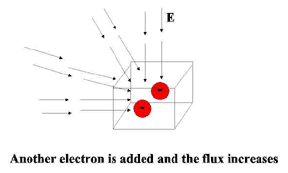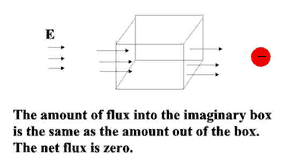Advertisements

# Physics Tutorial 1: Gauss’s Law & Conclusion

### Gauss’s Law

Gauss’s law is an alternative to Coulomb’s law for describing the relationship between electric charge and electric field. Gauss’s law can be modeled using an imaginary closed surface, referred to as a Gaussian surface. This model even helps us in understanding electromagnetism, which will be covered in a later tutorial.
First, we start by completely enclosing an electric charge with an imaginary surface or imaginary box. Let’s pretend this box has no effect on the electric field produced by the electric charge enclosed inside. Then, we look at the electric field at different points on this imaginary surface.
Since we now know that an electric charge produces an electric field, we could map out a three-dimensional electric field outside the box. Since there is a negative charge inside the box, the electric field would point toward the charge and into the box.
If there had been a positive charge inside the box, the electric field would point out of the box. This electric field mapping is called flux. Flux can be thought of as a flow into or out of the imaginary box, even though an electric field does not actually flow.
Now we place another negative electric charge inside the imaginary box. The amount, or magnitude, of the negative charge would increase and so does the flux.What happens if a negative charge and positive charge of the same magnitude are enclosed in the box? Both generate electric fields, one into the box and one out of the box, so that the net flux (net flow) is zero. Will charges outside the box give a net flux through the box? No, because the flux will flow through one half of the box and flow out the other half.There are two things you should note about flux, or the net flow through the Gaussian surface.
1. The net flow of electric field through the Gaussian surface depends on the net amount of electric charge contained within the surface.
2. The net flow through the surface is the product of the surface area and the component of electric field vector perpendicular to the surface. Think of it like a liquid flow- if the surface area was larger, more flux would flow into it and the net flux increases.
The above two points summarize Gauss’s law. Gauss’s law states that the total electric flux through a closed surface is proportional to the total electric charge enclosed within that surface. We can use this law to calculate fields caused by charge distributions with various symmetry properties. Gauss’s law is valid for any distribution of charges and for any closed surface.
So what good is this Gauss’s law, anyway? Let me give you an example. What if we were to place excess electric charge on a solid conductor that is at rest (electrostatic-no net motion of charge), and wanted to determine where those excess charges reside. Gauss’s law tells us that the excess charge would reside entirely on the surface, and not in the interior of the conductor material. Remember- this is only true when the solid conductor is electrostatic, and there is no net electric charge motion (current) of the ions and valence electrons that make up the conductor.
Here’s the proof. In an electrostatic situation the net effect of the electric field, , at every point of the solid conductor must be zero, or there would be current flowing. Now create an imaginary Gaussian surface inside the solid conductor. Since the net effect of is zero everywhere inside the conductor, Gauss’s law tells us the net flow into the Gaussian surface must be zero and that there is no excess charge inside the Gaussian surface.
Now continue shrinking the Gaussian surface like a collapsing balloon until it encloses a region of the solid conductor so small we can call it a point inside the conductor. The electric charge at that point must be zero. We can do this over and over at any point inside the conductor, and always determine that there is no excess electric charge inside the solid conductor. Therefore, the excess electric charge must reside on the outside of the electrostatic conductor. Wow!

### Summary

Tool One: Electrostatics and Coulomb’s law
1. An electric charge can be a single proton or electron, or a net electric charge, such as a group of free valence electrons in a conductor.
2. The interaction is called an electric force, .
3. Electric force can be described as a single interaction, or a net result.
4. Electrostatics is a description of interaction of electric charges at rest. Electric charge in motion is electric current. Electric current is in motion, and therefore is not electrostatic.
5. Electric interaction can also be modeled as an electric field, . The relationship between electric field, , and electric force, , can be characterized as

E
6. Coulumb’s Law quantifies the interaction of electric charges at rest.
Tool Two: Gauss’s law
1. Gauss’s law is an alternative to Coulomb’s law for describing the relationship between electric charge and electric field
2. Gauss’s law simplifies the electric field model by representing it as a quantity of net flow or flux through an imaginary Gaussian surface.
3. The net flow of electric field through the Gaussian surface depends on the net amount of electric charge contained within the surface.
4. The net flow through the surface is the product of the surface area and the component of electric field vector perpendicular to the surface. Think of it like a liquid flow- if the surface area was larger, more flux would flow into it and the net flux increases.
5. Gauss’s law does indeed have practical applications
Further References:

http://webphysics.davidson.edu/physlet_resources/bu_semester2/index.html

http://courses.science.fau.edu/~rjordan/phy2044/rev_notes.htm

http://ephysics.physics.ucla.edu/MIT/gausslaw/HTML/gausslaw.htm

Author is Assistant Professor of Physics at Modasa Science College, Gujarat, India.

### Training & Capacity Building In Physics

During 31st-Oct,2011 to 5th-Nove,2011, one week workshop ‘Training & Capacity building in Physics’ among the College Teachers was held. In this programme eminent Resource Person enlighten with various topics of which. The ppt of some of the presentations are as follow.

### Medicinal Plants By Guest, Prof. Krishna Rajput

Please leave ur comments & suggestions.